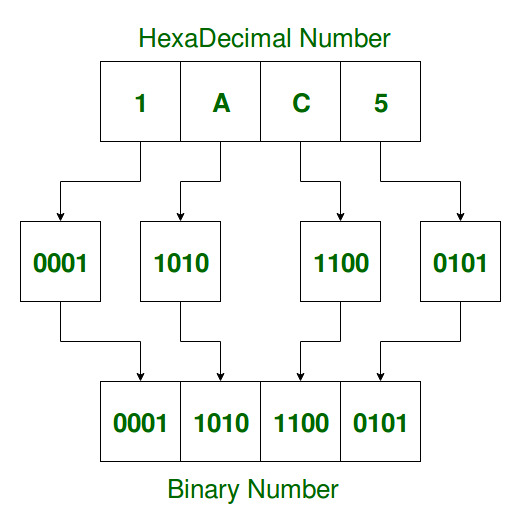# Java Program to Convert Hexadecimal to Binary

• Last Updated : 14 Aug, 2021

The Hexadecimal is a very useful Number System based on the premise of clubbing together 4 bits at a time to constitute a single entity of the system which is composed of 16 symbols including 10 digits ranging from 0-9 and the first six alphabets from A-F. The word Hexadecimal is derived from the words Hex which means six and decimal which means ten

Thus the combined word denotes sixteen which is six and ten added together. The Hexadecimal Sequences are also referred to as the base or radix 16. While dealing with different Number Systems, it becomes essential to be able to convert them from one system to another. In this article, we focus on converting Hexadecimal to Binary, which is a system comprised of 1’s and 0’s and is the mechanism through which the computers store and process instructions as well as data.

Examples :

```Hexadecimal Sequence : 458A
Binary Equivalent : 0100010110001010
Explanation : Binary representation of A : 1010
Binary representation of 8 : 1000
Binary representation of 5 : 0101
Binary representation of 4 : 0100

Binary Equivalent : 101100110110```There are two approaches to convert Hexadecimal to Binary and they are mentioned as follows :

1. Using the key-value pair for the corresponding conversion from the Hexadecimal character to its Binary equivalent
2. Converting the Hexadecimal to its Decimal equivalent which is further converted to its Binary equivalent

Approach 1 :

Using this approach, we formulate key-value and extracting every character of the Hexadecimal string, add its corresponding binary sequence and return the complete binary sequence.

1. Create a HashMap to store the key-value pairs.
2. Accept the Hexadecimal sequence as a string and extract each character while iterating through the length of the string.
3. Check if the extracted character is present in the keys of the HashMap.
4. If it is present, concatenate the string storing the binary sequence with the corresponding value of the key.
5. If it is not present, return Invalid Hexadecimal String.

Code:

## Java

 `// Java program to convert Hexadecimal to Binary` `import` `java.util.HashMap;` `class` `GFG {` `    ``// declaring the method to convert``    ``// Hexadecimal to Binary``    ``String hexToBinary(String hex)``    ``{` `        ``// variable to store the converted``        ``// Binary Sequence``        ``String binary = ``""``;` `        ``// converting the accepted Hexadecimal``        ``// string to upper case``        ``hex = hex.toUpperCase();` `        ``// initializing the HashMap class``        ``HashMap hashMap``            ``= ``new` `HashMap();` `        ``// storing the key value pairs``        ``hashMap.put(``'0'``, ``"0000"``);``        ``hashMap.put(``'1'``, ``"0001"``);``        ``hashMap.put(``'2'``, ``"0010"``);``        ``hashMap.put(``'3'``, ``"0011"``);``        ``hashMap.put(``'4'``, ``"0100"``);``        ``hashMap.put(``'5'``, ``"0101"``);``        ``hashMap.put(``'6'``, ``"0110"``);``        ``hashMap.put(``'7'``, ``"0111"``);``        ``hashMap.put(``'8'``, ``"1000"``);``        ``hashMap.put(``'9'``, ``"1001"``);``        ``hashMap.put(``'A'``, ``"1010"``);``        ``hashMap.put(``'B'``, ``"1011"``);``        ``hashMap.put(``'C'``, ``"1100"``);``        ``hashMap.put(``'D'``, ``"1101"``);``        ``hashMap.put(``'E'``, ``"1110"``);``        ``hashMap.put(``'F'``, ``"1111"``);` `        ``int` `i;``        ``char` `ch;` `        ``// loop to iterate through the length``        ``// of the Hexadecimal String``        ``for` `(i = ``0``; i < hex.length(); i++) {``            ``// extracting each character``            ``ch = hex.charAt(i);` `            ``// checking if the character is``            ``// present in the keys``            ``if` `(hashMap.containsKey(ch))` `                ``// adding to the Binary Sequence``                ``// the corresponding value of``                ``// the key``                ``binary += hashMap.get(ch);` `            ``// returning Invalid Hexadecimal``            ``// String if the character is``            ``// not present in the keys``            ``else` `{``                ``binary = ``"Invalid Hexadecimal String"``;``                ``return` `binary;``            ``}``        ``}` `        ``// returning the converted Binary``        ``return` `binary;``    ``}` `    ``// Driver Code``    ``public` `static` `void` `main(String[] args)``    ``{` `        ``// instantiating the class``        ``GFG ob = ``new` `GFG();``      ` `        ``String hex = ``"deafa"``;``      ` `        ``System.out.println(hex.toUpperCase());``      ` `        ``// printing and calling the``        ``// hexToBinary() function``        ``System.out.println(ob.hexToBinary(hex));``    ``}``}`

Output

```DEAFA
11011110101011111010```

Approach 2:

This approach first converts the Hexadecimal String into its decimal equivalent which is further converted into its binary equivalent. We make use of two functions, the first one to convert the Hexadecimal to Decimal and the second one to convert Decimal to Binary.

1. First, we write the function to convert the Decimal to Binary.
2. Next, we, write the function to convert Hexadecimal to Decimal and call the above function inside this function to convert the converted Decimal further into Binary.
3. In this function, we iterate through the length of the Hexadecimal String and extract every character one at a time.
4. Next, we check if the extracted characters are in the range 0-9 or A-F.
5. If the characters are present in the above-mentioned range we concatenate them to the decimal string.
6. Next, the decimal string is converted to Binary using the decimalToBinary() function.
7. If even one of the characters is not present in the above-mentioned range, Invalid Hexadecimal String is returned as the output.

Code:

## Java

 `// Java program to convert Hexadecimal to Binary` `class` `GFG {` `    ``// method to convert Decimal to Binary``    ``String decimalToBinary(``int` `decimal)``    ``{` `        ``// variable to store the converted``        ``// binary string``        ``String binaryString = ``""``;` `        ``// loop to generate the binary``        ``while` `(decimal != ``0``) {` `            ``// concatenating the remainder``            ``// on dividing by 2 to the``            ``// binary string``            ``binaryString = (decimal % ``2``) + binaryString;` `            ``// updating the decimal integer``            ``// by dividing by 2 in each iteration``            ``decimal /= ``2``;``        ``}` `        ``// loop to ensure that each``        ``// Hexadecimal character is``        ``// represented by 4 bits``        ``while` `(binaryString.length() % ``4` `!= ``0``) {``            ``// adding leading 0's if the``            ``// character is represented by less``            ``// than 4 bits``            ``binaryString = ``"0"` `+ binaryString;``        ``}` `        ``// returning the converted binary string``        ``return` `binaryString;``    ``}` `    ``// method to convert Hexadecimal to Binary``    ``String hexToBinary(String hexadecimal)``    ``{` `        ``// declaring the variables``        ``int` `i;``        ``char` `ch;``        ``String binary = ``""``;``        ``int` `returnedBinary;` `        ``// converting the accepted Hexadecimal``        ``// String to upper case``        ``hexadecimal = hexadecimal.toUpperCase();` `        ``// loop to iterate through the length``        ``// of the Hexadecimal String``        ``for` `(i = ``0``; i < hexadecimal.length(); i++) {` `            ``// extracting the characters``            ``ch = hexadecimal.charAt(i);` `            ``// condition to check if``            ``// the character is not a valid Hexadecimal``            ``// character``            ``if` `(Character.isDigit(ch) == ``false``                ``&& ((``int``)ch >= ``65` `&& (``int``)ch <= ``70``)``                       ``== ``false``) {` `                ``// returning Invalid Hexadecimal``                ``// String for the invalid Hexadecimal``                ``// character``                ``binary = ``"Invalid Hexadecimal String"``;``                ``return` `binary;``            ``}` `            ``// checking if the character is a valid``            ``// Hexadecimal alphabet``            ``else` `if` `((``int``)ch >= ``65` `&& (``int``)ch <= ``70``)` `                ``// converting alphabet to``                ``// corresponding value such as 10``                ``// for A and so on using ASCII code``                ``returnedBinary = (``int``)ch - ``55``;``            ``else``                ``returnedBinary``                    ``= Integer.parseInt(String.valueOf(ch));` `            ``// converting the decimal to binary``            ``// by calling the decimalToBinary() method``            ``binary += decimalToBinary(returnedBinary);``        ``}` `        ``// returning the converted binary sequence``        ``return` `binary;``    ``}` `    ``// Driver Code``    ``public` `static` `void` `main(String[] args)``    ``{` `        ``// instantiating the class``        ``GFG ob = ``new` `GFG();``        ` `        ``String hex = ``"abcfde"``;``      ` `        ``System.out.println(hex);``      ` `        ``// printing and calling the``        ``// hexToBinary() function to display the``        ``// output``        ``System.out.println(ob.hexToBinary(hex));``    ``}``}`

Output

```abcfde
101010111100111111011110```

My Personal Notes arrow_drop_up